# E-PolyLearning

 1. Choose the set of figures which follows the given rule. Rule: Closed figures losing their sides and open figures gaining their sides.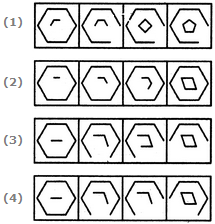a. 1 b. 2 c. 3 d. 4

 2. Choose the set of figures which follows the given rule. Rule: Any figure can be traced by a single unbroken line without retracting.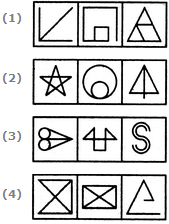a. 1 b. 2 c. 3 d. 4
 3. Choose the set of figures which follows the given rule. Rule: Closed figures gradually become open and open figures gradually become closed.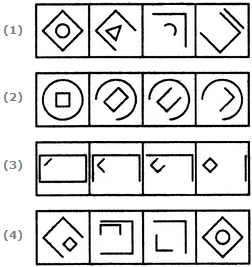a. 1 b. 2 c. 3 d. 4
 4. Choose the set of figures which follows the given rule. Rule: Closed figures become more and more open and open figures become more and more closed.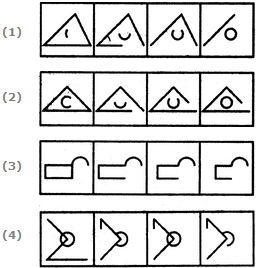a. 1 b. 2 c. 3 d. 4
 5. Choose the set of figures which follows the given rule. Rule: The series becomes complex as it proceeds.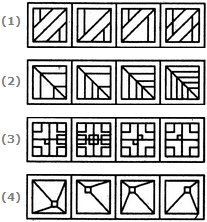a. 1 b. 2 c. 3 d. 4